### Home > GC > Chapter 10 > Lesson 10.3.1 > Problem10-96

10-96.

For each pair of triangles below, decide if the pair is similar, congruent or neither. Justify your conclusion (such as with a similarity or congruence property like $\text{AA}∼$ or $\text{SAS}∼$ or the reasons why the triangles cannot be similar or congruent). Assume that the diagrams are not drawn to scale.

1.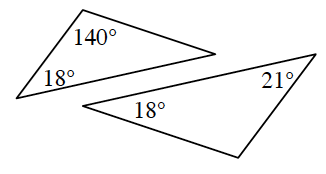Is the last angle congruent? Use the Triangle Angle Sum Theorem to find out.

Not similar.
There are not three pairs of corresponding angles that are congruent.

1.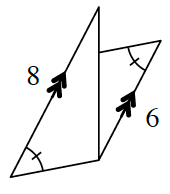What kind of angles do parallel lines create? Does this make the triangles similar?

1.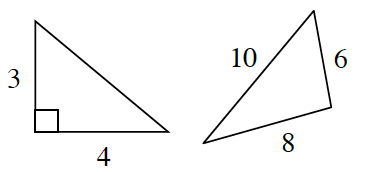Find the missing side length. Are the side ratios equal?

1.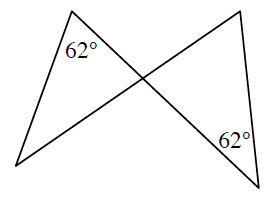What kind of angles are these?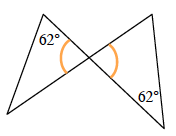Similar by $\text{AA}∼$.